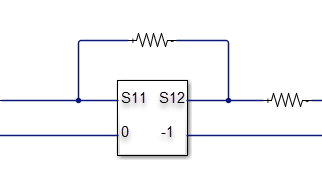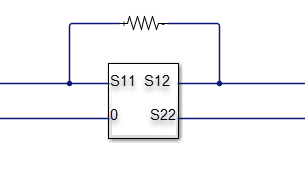# computeBudget

Compute results of RF budget object

## Syntax

``computeBudget(rfobj)``

## Description

example

````computeBudget(rfobj)` computes the result of an RF budget object. You can use this method only when the AutoUpdate property of the RF budget object is set to `false`.```

## Examples

collapse all

Create a modulator with output-referred second-order intercept set to 20 and available power gain set to 3.

`m = modulator(OIP2=20,Gain=3,ImageReject=false,ChannelSelect=false);`

Create an amplifier with 10 dB gain.

`a = amplifier(Gain=10);`

Create an RF budget object specifying the input frequency of the signal, power applied at cascade, and signal bandwidth.

`b1 = rfbudget([m a],2.1e9,-30,100e6,AutoUpdate=false)`
```b1 = rfbudget with properties: Elements: [1x2 rf.internal.rfbudget.RFElement] InputFrequency: 2.1 GHz AvailableInputPower: -30 dBm SignalBandwidth: 100 MHz Solver: Friis AutoUpdate: false ```

Use the `computeBudget` function to compute the results of an RF budget object.

`computeBudget(b1)`

Display the RF budget results.

`b1`
```b1 = rfbudget with properties: Elements: [1x2 rf.internal.rfbudget.RFElement] InputFrequency: 2.1 GHz AvailableInputPower: -30 dBm SignalBandwidth: 100 MHz Solver: Friis AutoUpdate: false Analysis Results OutputFrequency: (GHz) [ 3.1 3.1] OutputPower: (dBm) [ -27 -17] TransducerGain: (dB) [ 3 13] NF: (dB) [ 0 0] IIP2: (dBm) [] OIP2: (dBm) [] IIP3: (dBm) [ Inf Inf] OIP3: (dBm) [ Inf Inf] SNR: (dB) [63.98 63.98] ```

## Input Arguments

collapse all

RF budget object, specified as a `rfbudget` object.

## Algorithms

collapse all

### S21 = 0

If S21 of an element is zero, you make the following modifications to that element:

• S21 = 0 and S11 = -1• S21 = 0 and S22 = -1• S21 = 0, S22 = -1, and S = -1• S21 = 0## Version History

Introduced in R2017a